# Precalculus : Trigonometric Functions

## Example Questions

1 2 10 11 12 13 14 15 16 18 Next →

### Example Question #57 : Fundamental Trigonometric Identities

Which of the following trigonometric identities is INCORRECT?

Possible Answers: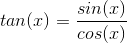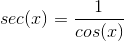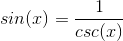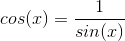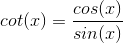Correct answer:Explanation:

Cosine and sine are not reciprocal functions.and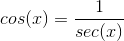### Example Question #58 : Fundamental Trigonometric Identities

Using the trigonometric identities prove whether the following is valid: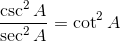Possible Answers:

False

Uncertain

True

Only in the range of: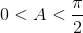Only in the range of: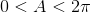Correct answer:

True

Explanation:

We begin with the left-hand side of the equation and utilize basic trigonometric identities, beginning with converting the inverse functions to their corresponding base functions: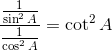Next, we rewrite the fractional division in order to simplify the equation: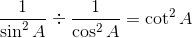In fractional division we multiply by the reciprocal as follows: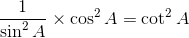If we reduce the fraction using basic identities we see that the equivalence is proven: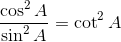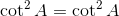### Example Question #59 : Fundamental Trigonometric Identities

Which of the following identities is incorrect?

Possible Answers: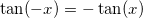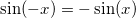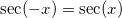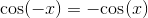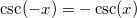Correct answer:Explanation:

The true identity is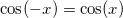because cosine is an even function.

### Example Question #60 : Fundamental Trigonometric Identities

State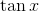in terms of sine and cosine.

Possible Answers: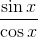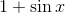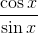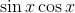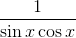Correct answer:Explanation:

The definition of tangent is sine divided by cosine.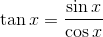### Example Question #61 : Fundamental Trigonometric Identities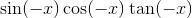Possible Answers: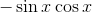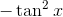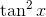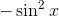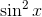Correct answer:Explanation:

Using these basic identities: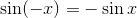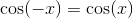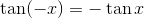we find the original expression to be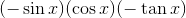which simplifies to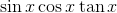.

Further simplifying: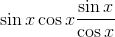The cosines cancel, giving us### Example Question #62 : Fundamental Trigonometric Identities

Which of the following is the best answer for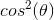?

Possible Answers: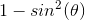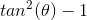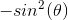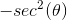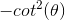Correct answer:Explanation:

Write the Pythagorean identity.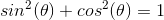Substract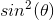from both sides.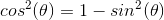The other answers are incorrect.

### Example Question #63 : Fundamental Trigonometric Identities

Express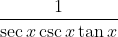in terms of only sines and cosines.

Possible Answers: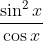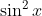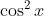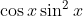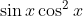Correct answer:Explanation:

The correct answer is. Begin by substituting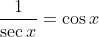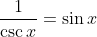, and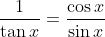. This gives us: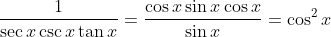.

### Example Question #171 : Trigonometric Functions

Express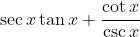in terms of only sines and cosines.

Possible Answers: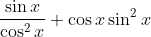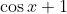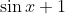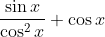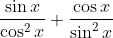Correct answer:Explanation:

To solve this problem, use the identities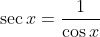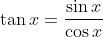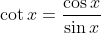, and. Then we get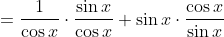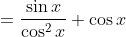1 2 10 11 12 13 14 15 16 18 Next →

### All Precalculus Resources